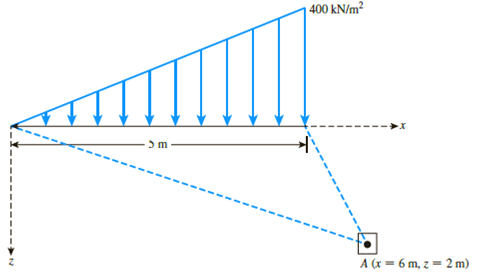Chapter 10, Problem 10.22PPrinciples of Geotechnical Enginee...

9th Edition
Braja M. Das + 1 other
ISBN: 9781305970939

Solutions

Chapter
SectionPrinciples of Geotechnical Enginee...

9th Edition
Braja M. Das + 1 other
ISBN: 9781305970939
Textbook Problem

Refer to Figure 10.49. For the linearly increasing vertical loading on an infinite strip of width 5 m, determine the vertical stress increase, Δσz, at A.Figure 10.49

To determine

Calculate the increase in vertical stress at A.

Explanation

Given information:

The width of the finite strip (B) is 5m.

The location of point A (x,z) is (6m,2m).

The intensity of loading (q) is 400kN/m2.

Calculation:

Sketch the linearly increasing vertical loading on finite strip as shown in Figure 1.

Refer to Figure 1.

Calculate the angle (α1) as shown below.

tanα1=26α1=tan1(13)α1=18.43°

Calculate the angle (α2) as shown below.

tanα1=21α1=tan1(2)α1=63.43°

Calculate the angle (δ) as shown below.

tanδ=12δ=tan1(12)δ=26.565°

Calculate the angle (α) as shown below.

α=α2α1

Substitute 63

Still sussing out bartleby?

Check out a sample textbook solution.

See a sample solution

The Solution to Your Study Problems

Bartleby provides explanations to thousands of textbook problems written by our experts, many with advanced degrees!

Get Started

Give examples of rewards that one gains from doing volunteer work.

Engineering Fundamentals: An Introduction to Engineering (MindTap Course List)

Are top managers likely to be more effective if they have IT experience? Why or why not?

Systems Analysis and Design (Shelly Cashman Series) (MindTap Course List)

Determine the maximum load in the weld in kips per inch of length.

Steel Design (Activate Learning with these NEW titles from Engineering!)

Why must a mushroomed chisel or hammer be reground?

Welding: Principles and Applications (MindTap Course List)